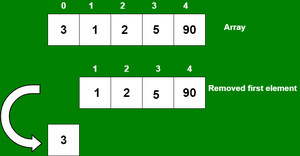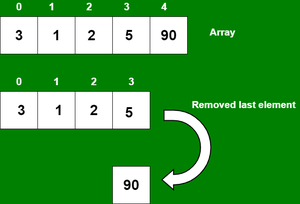# How to delete an element from an array using JavaScript ?

• Last Updated : 21 Dec, 2021

In PHP, the array is a type of data structure that allows us to store similar types of data under a single variable. The array is helpful to create a list of elements of similar types, which can be accessed using their index.  In this article, we will discuss different ways to remove elements from the array. There are many methods that are used to remove elements from the PHP array which are discussed below:

We can delete an element or item from an array using two functions which is:

Remove Array elements by Using array_shift() function: This function is used to delete one or more than one element from the array and these elements get deleted from the beginning of the array and because of the deleted elements or items from the array, the length of the array also gets decreased by the number of elements deleted from the array. After deleting the elements, we use the print_r() function to print the array information.

Below are examples of how to do this: Let’s see how to delete an element at the beginning of the array using the array_shift() function.

```Input:  arr[] = {3, 1, 2, 5, 90}, size = 5, capacity = 5
Output: arr[] = {_, 1, 2, 5, 90}, size = 4, capacity = 5```

Example 1: In this example, we use an indexed array to delete elements at the beginning of an array using the array_shift() function.## PHP

 ``

Output:

`Array (  => 1  => 2  => 5  => 90  )`

Example 2: In this example, we use an associative array to delete elements at the beginning from an array using the array_shift() function.

## PHP

 ` 3,  ` `      ``"second"` `=> 1,  ` `      ``"third"` `=>  2, ` `      ``"fourth"` `=> 5, ` `      ``"fifth"` `=> 90 ` `); ` `   `  `array_shift``(``\$arr``); ` `print_r(``\$arr``); ` `   `  `?>`

Output:

`Array ( [second] => 1 [third] => 2 [fourth] => 5 [fifth] => 90 )`

Remove Array elements by Using array_pop() function: This function is used to pop or delete elements or items from an array. We can pop one or more than one element from the array and these elements get deleted from the end of the array and because of the popped elements from the array, the length of the array also gets decreased by the number of elements popped from the array. After deleting the elements, we use the print_r() function to print the array information.

Below are examples of how to do it: Let’s see how to delete an element at the end of the array using the array_pop() function.

```Input:  arr[] = {3, 1, 2, 5, 90}, size = 5, capacity = 5
Output: arr[] = {3, 1, 2, 5, _}, size = 4, capacity = 5```

Example 1: In this example, we use an indexed array to delete elements at the end from an array using the array_pop() function.## PHP

 ``

Output:

`Array (  => 3  => 1  => 2  => 5 )`

Example 2: In this example, we use an associative array to delete elements at the end from an array using the array_pop() function.

## PHP

 ` 3,  ` `      ``"second"` `=> 1,  ` `      ``"third"` `=>  2, ` `      ``"fourth"` `=> 5, ` `      ``"fifth"` `=> 90 ` `); ` `   `  `array_pop``(``\$arr``); ` `print_r(``\$arr``); ` `   `  `?>`

Output:

`Array ( [first] => 3 [second] => 1 [third] => 2 [fourth] => 5 )`

My Personal Notes arrow_drop_up
Recommended Articles
Page :